# Symbols

## Mathematical notation is sometimes unclear

Mathematical expressions with their operators and symbols form the basis for algebra and calculus. But this notation can sometimes be ambiguous, as the following examples illustrate:

• In $a(b+c)$, is symbol $a$ a variable or a function?
• Is $ab$ the multiplication of $a$ and $b$, or is $ab$ a single symbol?
• In expression $3ms$, is $m$ the symbol for meters or a variable? Is $s$ the symbol for seconds? Or is $ms$ the symbol for milliseconds?
• In $3e^2$, is $e$ a variable or Euler's constant?

For students, the meaning of symbols is usually clear from the context. For Algebrakit, it is up to you to provide this information.

## Confirming symbols

When you preview an exercise, Algebrakit will automatically attempt to establish what the symbols mean and ask you to confirm these interpretations.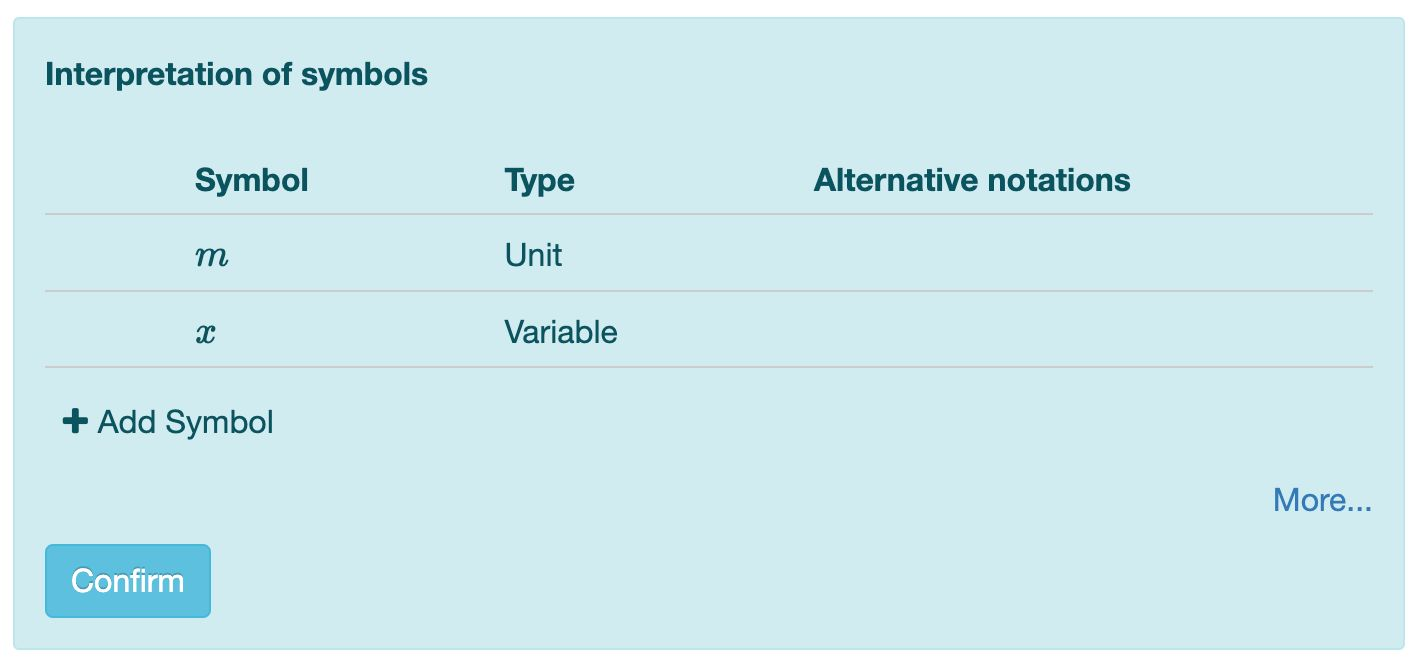Figure: Confirm symbol interpretations

You can adjust the suggested interpretations if needed. After confirming, Algebrakit will store the symbol interpretations in the exercise, so you won't have to verify them again.

Warning

Make sure to always preview an exercise before saving it. Algebrakit will only run exercises with confirmed symbols.

## The symbols panel

You can see all symbol interpretations in the Symbols tab, which you can find in the top bar.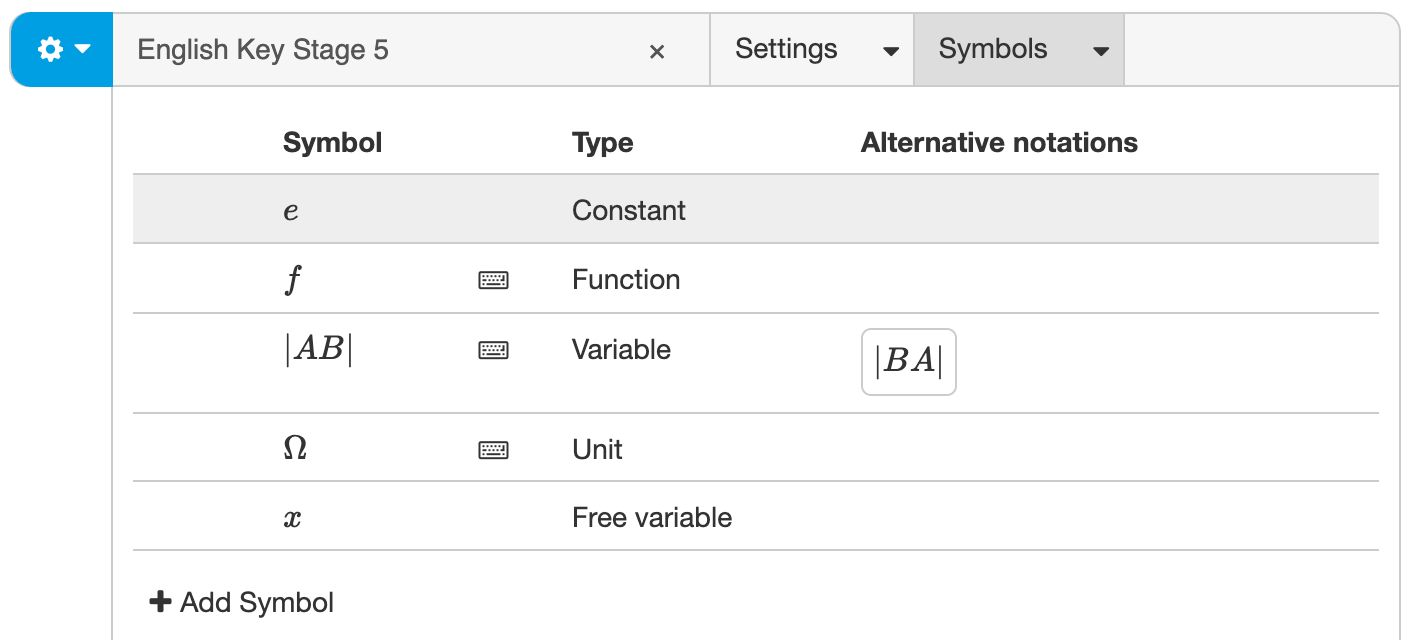Figure: The Symbols panel

Use the button to add a new symbol or click an item in the list to adjust it. You can configure the symbol name, symbol type, and alternative notations. You can also specify if the symbol must be visible in the formula editor when the exercise starts.

### Symbol name

Mathematical symbols are often straightforward, like $x$, $y$, $t$, etc. But this is not always the case. For example, expressions with complex units or variable names occur frequently in geometry or STEM.

Algebrakit allows you to declare symbols that can have any of the following characteristics:

• multi-letter symbols, like $ab$ or $meters$
• symbols with special characters, like $\Omega$, $\angle A$, or $|AB|$
• symbols with subscript or superscript, like $x_0$ or $x^a$
• symbols with modifiers, like $\tilde{x}$, $\bar{xy}$, or $\hat{a}$.
• word symbols, like '$\mathrm{revenue}$' or '$\mathrm{total\ price}$'.
• any other character sequence, like $+^{\%}$, or $sin(x)$.
But be careful: a symbol will replace any previous meaning it might have had. For example, if you declare a variable $sin(x)$, it loses its definition as a trigonometric function.

Example: An exercise using angles as variables.

### Symbol type

A symbol can have any of the following types:

• Variable. You can use variables in equations, function definitions, differentiation, etc. Algebrakit writes variables in italics.
• Unit. If the symbol represents a standard unit, like kilogram (kg) or yards (yd), Algebrakit will also correctly interpret alternative notations. For example, $\mathrm{meter}$, $\mathrm{meters}$ and $\mathrm{m}$ are all interpreted as the same unit. However, you can also define a custom unit, such as $\mathrm{apples}$. Algebrakit writes units in a regular (non-italic) font.
• Constant. Use this for symbols representing a predetermined value. The only constant that currently needs to be declared is Euler's constant $e$. You don't have to define $\pi$.
• Function. Declare a symbol as a function to guarantee that Algebrakit interprets the parentheses as function notation. For example, declare $a$ as a function to interpret $a(x+2)$ as a function application. If $a$ is a variable, then $a(x+2)$ is interpreted as $a\times(x+2)$.
• Probability function. $P$ is the usual symbol for probability, such a $P(X\ge 2)$. However, you can define a different symbol.
• Free variable. If you define a symbol a free variable, then students can decide their variable name. Currently, students are not allowed to choose multi-letter names.

Example: An exercise using free variables.

#### Singular and plural forms of units

Units are sometimes written differently depending on the number. For example, you write 2 apples and 1 apple. If the exercise is randomized, which we will discuss later, you do not know in advance if you should use the singular or plural form. You can add the singular form and let Algebrakit automatically choose the correct format.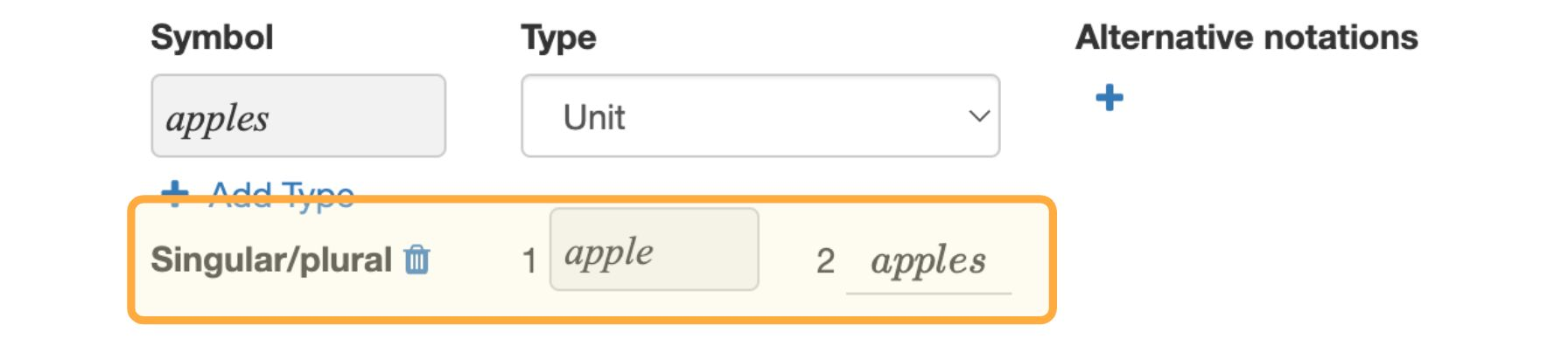These images are from an oversimplified and nonrandom example to illustrate the case. We have defined the symbol '$\mathrm{apples}$' a unit with '$\mathrm{apple}$' as its singular form. Note how we used the plural form in the question text. Algebrakit replaced it by the singular form.You can see in the exercise below that Algebrakit adjusts the number of the unit automatically. Students are allowed to use both forms in their answers.

Figure: Declaring a singular form for a unit

Note

Defining a singular form is only possible if the symbol type is 'unit' and if the symbol does not have a single-letter name.

### Alternative notations

Sometimes different symbols mean the same thing. Some examples are:

• Words and symbols: $2m = 2\ \mathrm{meter} = 2\ \mathrm{meters}$
• (The length of) line segments: $|AB|=|BA|$
• Angles: $\angle ABC = \angle CBA$
• Triangles: $\Delta ABC = \Delta BCA = \Delta CAB$.

You can add such alternative notations to the symbol definition, allowing students to choose the symbol to use.

In the example below, a correct answer is $BC^2=AB^2+BC^2$. Thanks to alternative notations, students can also answer $CB^2=BA^2+BC^2$. Try it yourself.

### Adding symbols to the formula editor

It is convenient for students if variable and unit names are immediately available in the formula editor. This is especially true for more complex names, like $\angle B_1$ or $|BA|$, or when students work on a mobile device and cannot type with their keyboard.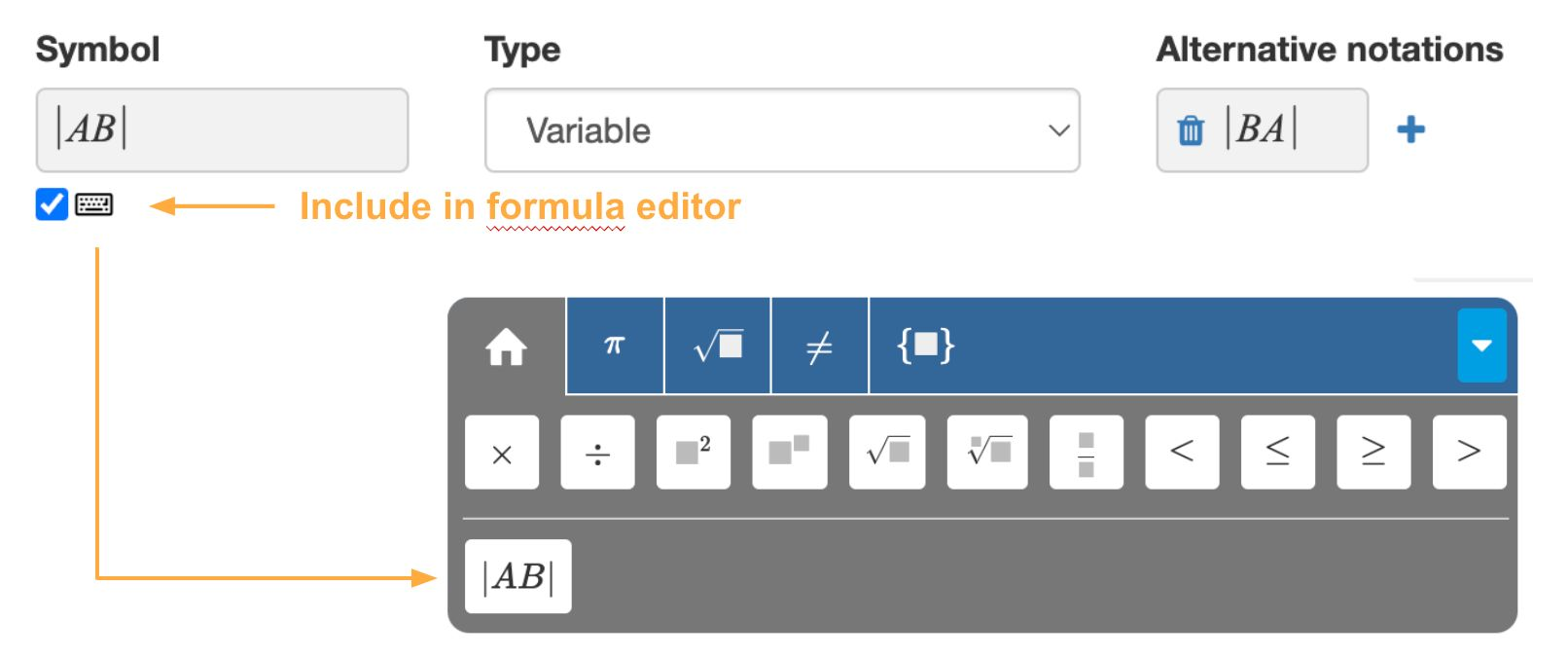Figure: Including symbols in the formula editor.

The formula editor will not include symbols that aren't used in the question.

### Symbols with multiple types

In some STEM exercises, the same symbol can have multiple meanings. An example is the following exercise:

"A force of 2700 N is exerted on a car of 1200 kg. Calculate the car's acceleration."

The image below shows a possible solution. Note that the symbol $m$ is not only used for the mass of the car but also the unit meter in the final answer.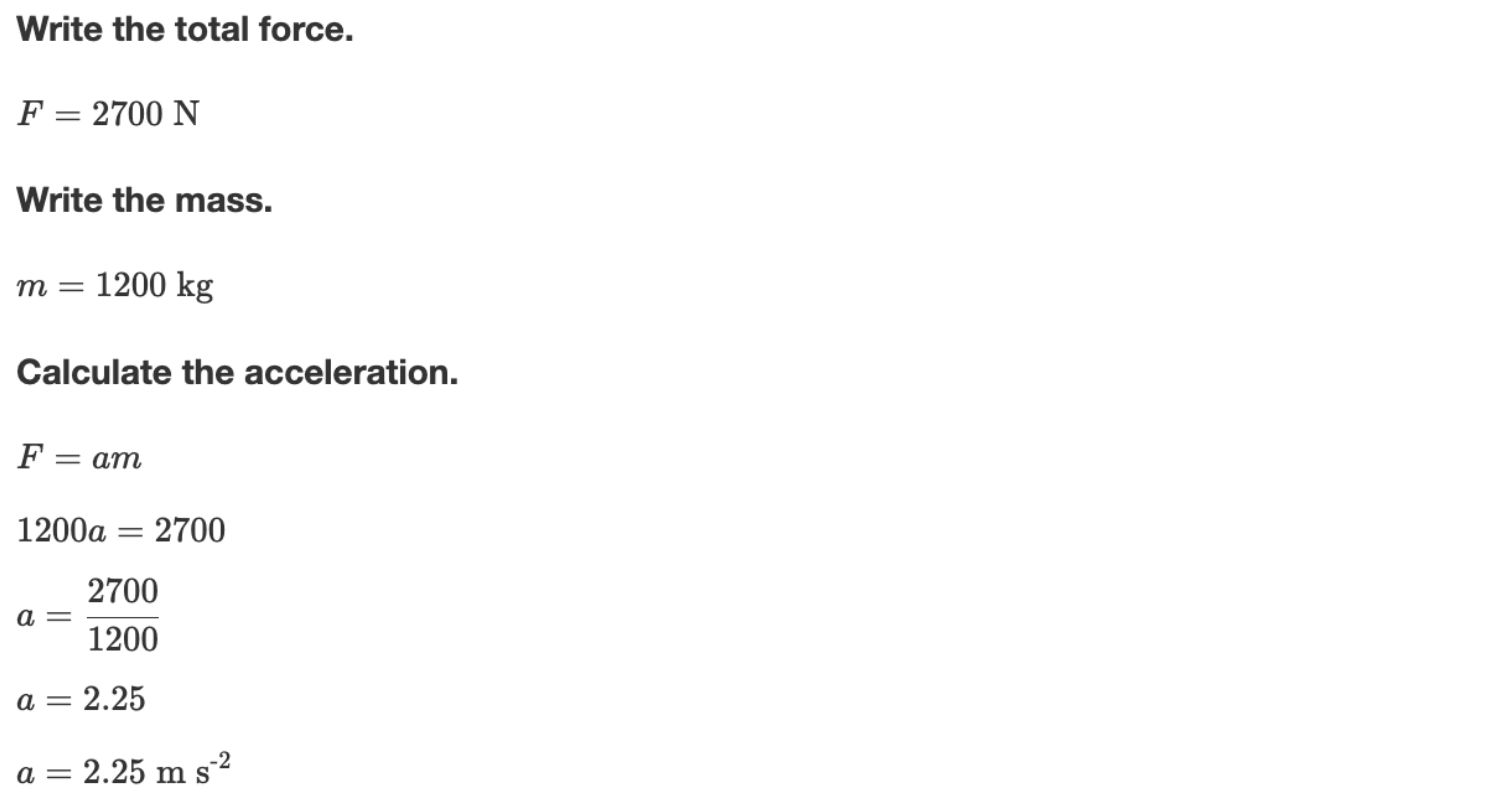Figure: The solution of the example problem.

Algebrakit will automatically determine what interpretation to use in the student's work. Authors have to be more specific, however.

In the image below, the symbol $m$ has two types: variable and unit. The author must use a unique symbol for each interpretation to prevent confusion. Algebrakit will always show the symbol $m$ and not these unique symbols when you run the exercise.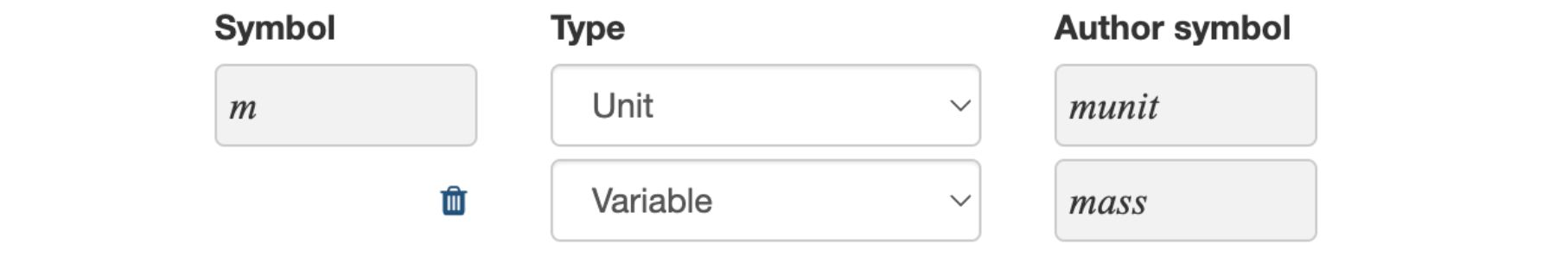Figure: Defining symbols with multiple meanings.

You can try this exercise below. Click on the Edit button to see how we created it.

## Word variables

Sometimes words or even small sentences are used as variables, especially in lower grades where students are learning the concept of variables.

An example is the expression: $\mathrm{total\ price} = 2.50\times\mathrm{total\ distance} + 14$.

To prevent Algebrakit from interpreting '$\mathrm{total\ price}$' as '$total\times price$', you must define it a variable.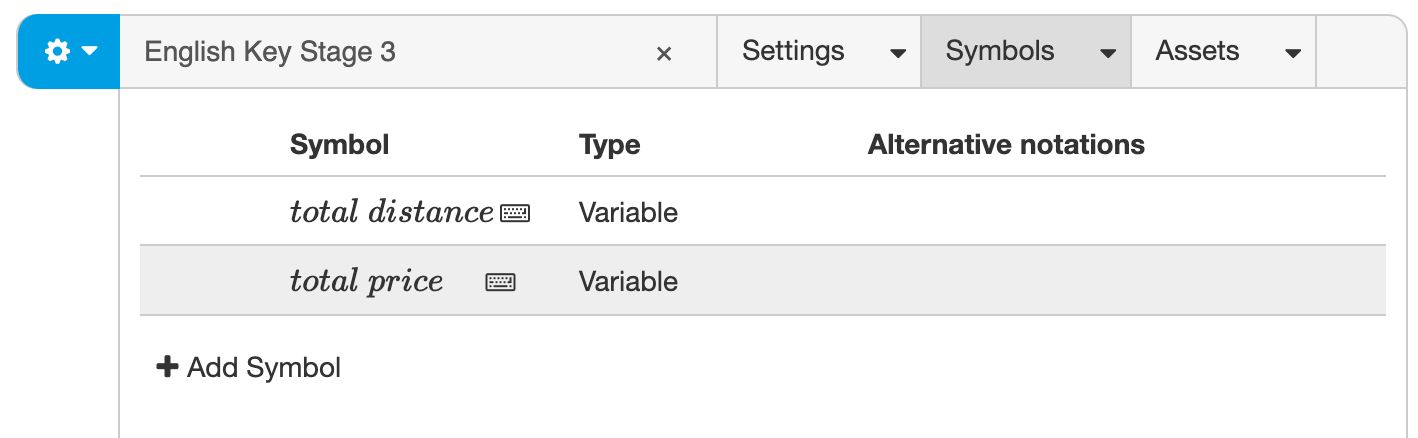Figure: Defining word variables.

## Special symbols

In some math problems, special symbols emerge in the solution. Examples are the discriminant in quadratic equations, the integration constant of anti-derivatives, the arbitrary integer in the general solutions of trigonometric equations and the symbols for basis vectors. You can configure what symbols Algebrakit uses in these situations.

Click "More..." in the bottom right of the symbols tab to open the form for special symbols.

### Discriminant

The discriminant is an expression used in the Quadratic Formula for quadratic equations. The symbol for the discriminant is usually $D$ or $d$.

$a x^2 + b x + c = 0 \Rightarrow x = \frac{-b \pm \sqrt{D}}{2a}, D=b^2-4ac$

You can override the symbol for the discriminant. For example, you can use the symbol $\Delta$ as follows: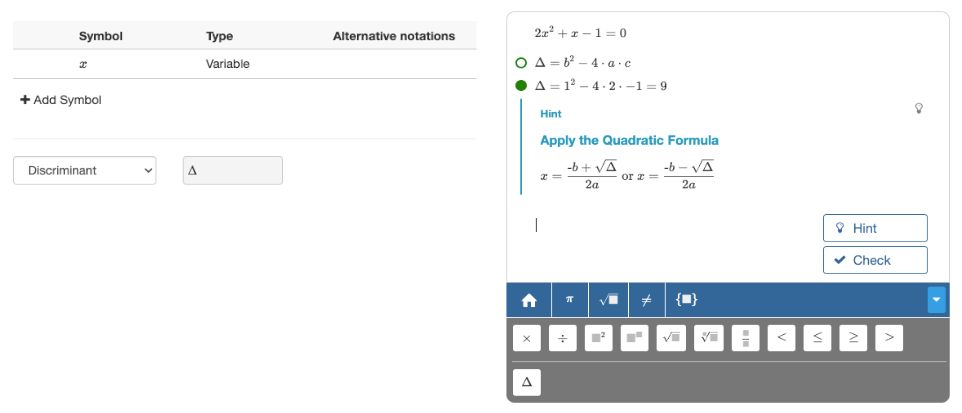Figure: Defining the symbol for the discriminant.

To do

Add examples for the other special symbols:

• Integration constant
• Constant for general solution in trigonometry
• Base vectors